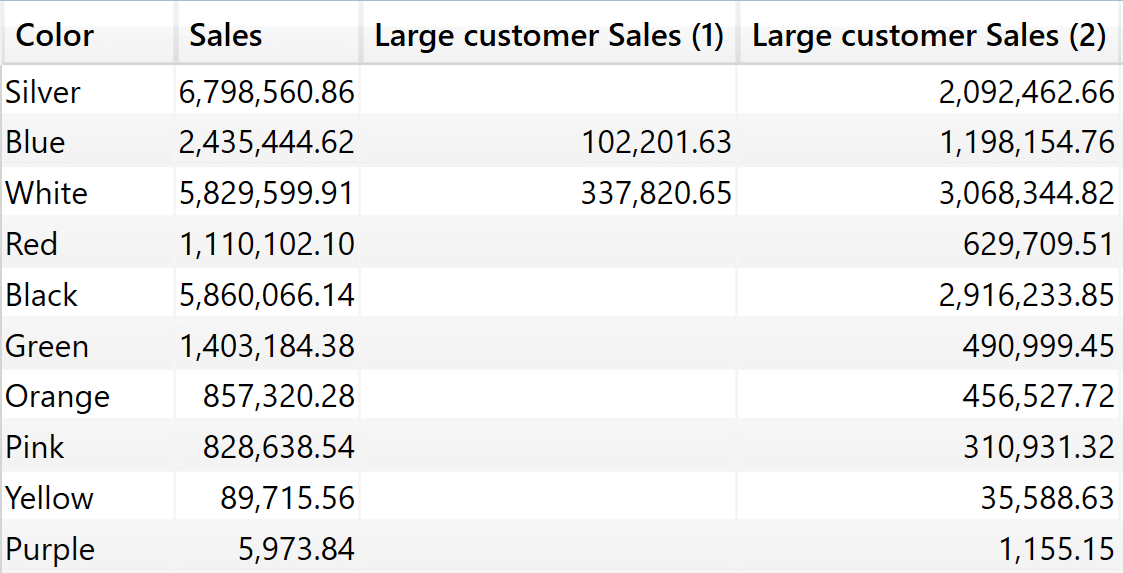Introduced in December 2020, the DEFINE COLUMN statement lets you define a calculated column local to a query. The column is not persisted in the model, it exists only for the lifetime of the query. Apart from that, it is a calculated column in every sense of the term.

The extension of DAX with the capability to define calculated columns local to a query is needed in order to support composite models over Analysis Services (AS). There are no limitations in the use of the feature. For this reason, you can take advantage of local columns in any DAX query. We refer to calculated columns defined in a query as query calculated columns, or query columns for short.

The syntax to define a query column is the following:

```DEFINE
COLUMN Sales[Sales Category] =
IF (
Sales[Net Price] >= 1000,
"High",
"Low"
)
EVALUATE
SUMMARIZECOLUMNS (
Sales[Sales Category],
"Amt", [Sales Amount]
)
```

Once the column is defined in the query, it can be used like any other column in the model. As you can see in the example query, SUMMARIZECOLUMNS can summarize by the Sales Category column, even though the column does not really exist in the model.

Being part of the DEFINE section of a query, a query calculated column can be used in multiple EVALUATE statements, as is the case for query variables, measures, and tables:

```DEFINE
COLUMN Sales[Sales Category] =
IF ( Sales[Net Price] >= 1000, "High", "Low" )

EVALUATE
SUMMARIZECOLUMNS (
Sales[Sales Category],
"Amt", [Sales Amount]
)

EVALUATE
SUMMARIZECOLUMNS (
'Product'[Brand],
Sales[Sales Category],
"Amt", [Sales Amount]
)
```

Query calculated columns are computed with no filter context, much like model calculated columns are. This is important to learn and remember from the very beginning. Indeed, you might be tempted to assume that the two following EVALUATE statements compute the same result, when in fact they do not:

```DEFINE
COLUMN 'Product'[Popular Product] =
IF ( [Sales Amount] >= 100000, "Popular", "Not Popular" )

EVALUATE
SUMMARIZECOLUMNS (
'Product'[Popular Product],
TREATAS ( { "CY 2007" }, 'Date'[Calendar Year] ),
"Amt", [Sales Amount]
)

EVALUATE
CALCULATETABLE (
SUMMARIZE (
'Product',
"@Popular Product", IF ( [Sales Amount] >= 100000, "Popular", "Not Popular" )
),
[@Popular Product],
"Amt", [Sales Amount]
),
TREATAS ( { "CY 2007" }, 'Date'[Calendar Year] )
)
```

Despite the code of both columns Product[Popular Product] and [@Popular Product] being the same, the [@Popular Product]  column computed inside ADDCOLUMNS is evaluated in the filter context of CALCULATETABLE which is filtering only one year. The Product[Popular Product] query column on the other hand, is computed outside of any filter context. Therefore, their definition of a product being popular or not is very different.

In order to obtain the same behavior, one would need to remove any filter from the filter context, except from the Product being iterated by ADDCOLUMNS to preserve the context transition:

```EVALUATE
CALCULATETABLE (
SUMMARIZE (
'Product',
"@Popular Product",
CALCULATE (
IF ( [Sales Amount] >= 100000, "Popular", "Not Popular" ),
ALLEXCEPT ( Sales, 'Product' )
)
),
[@Popular Product],
"Amt", [Sales Amount]
),
TREATAS ( { "CY 2007" }, 'Date'[Calendar Year] )
)
```

The reasoning depicted above leads to the conclusion that using query calculated columns with the sole purpose of simplifying code is a dangerous practice. Indeed, one might be tempted to use query calculated columns to simplify the following code:

```EVALUATE
SUMMARIZECOLUMNS (
'Product'[Color],
"Sales", [Sales Amount],
"Large customer sales",
CALCULATE (
[Sales Amount],
FILTER (
Customer,
[Sales Amount] >= 100000
)
)
)
```

It is tempting to replace the complex FILTER condition in Large customer Sales with the following code:

```DEFINE
COLUMN Customer[IsLarge] = [Sales Amount] >= 100000
EVALUATE
SUMMARIZECOLUMNS (
'Product'[Color],
"Sales", [Sales Amount],
"Large customer Sales",
CALCULATE (
[Sales Amount],
Customer[IsLarge] = TRUE
)
)
```

Unfortunately, the two queries lead to very different results, because of the semantics of query calculated columns:

```DEFINE
COLUMN Customer[IsLarge] = [Sales Amount] >= 100000
EVALUATE
SUMMARIZECOLUMNS (
'Product'[Color],
"Sales", [Sales Amount],
"Large customer Sales (1)",
CALCULATE (
[Sales Amount],
FILTER (
Customer,
[Sales Amount] >= 100000
)
),
"Large customer Sales (2)",
CALCULATE (
[Sales Amount],
Customer[IsLarge] = TRUE
)
)
```

The result clearly shows the different behaviors of the two formulas.We deliberately avoided talking about performance. At the time of writing, the feature is still in preview and we do not have enough experience with it to provide any best practice. Nonetheless, we noted that the query plan of query columns is different than that of columns added through ADDCOLUMNS. The two following queries produce a different query plan, despite being identical in their result:

```DEFINE
COLUMN Customer[IsLarge] = [Sales Amount] >= 100000
EVALUATE
Customer

EVALUATE
ADDCOLUMNS ( Customer, "IsLarge", [Sales Amount] >= 100000 )
```

The query with ADDCOLUMNS is slightly faster and it does not include any CallbackDataID. That said, this is by no means an indication about performance, because the feature is in preview and we still need to investigate the best practices in the usage of query columns.

Query columns have been introduced in DAX specifically to support composite models. In that respect, they do their job, letting a developer define a calculated column that is local to a query. Using query columns in regular queries is rather complex, because of the different semantics when compared with columns added with ADDCOLUMNS.

SUMMARIZECOLUMNSCreate a summary table for the requested totals over set of groups.

`SUMMARIZECOLUMNS ( [<GroupBy_ColumnName> [, [<FilterTable>] [, [<Name>] [, [<Expression>] [, <GroupBy_ColumnName> [, [<FilterTable>] [, [<Name>] [, [<Expression>] [, … ] ] ] ] ] ] ] ] ] )`

ADDCOLUMNSReturns a table with new columns specified by the DAX expressions.

`ADDCOLUMNS ( <Table>, <Name>, <Expression> [, <Name>, <Expression> [, … ] ] )`

CALCULATETABLEContext transition

Evaluates a table expression in a context modified by filters.

`CALCULATETABLE ( <Table> [, <Filter> [, <Filter> [, … ] ] ] )`

FILTERReturns a table that has been filtered.

`FILTER ( <Table>, <FilterExpression> )`